# FIFO Push Relabel Algorithm

• Last Updated : 08 Mar, 2022

The push–relabel algorithm (alternatively, pre flow–push algorithm) is an algorithm for computing maximum flows in a flow network. Push-relabel algorithms work in a more localized manner than the Ford Fulkerson method. Rather than examine the entire residual network to find an augmenting path, push-relabel algorithms work on one vertex at a time, looking only at the vertex’s neighbors in the residual network.

Intuition

The push relabels algorithm can be understood in terms of fluid flows. All vertex represent pipe junctions and all directed edges represent pipes having a specific capacity. Each vertex has two special properties. They contain a reservoir to store any excess flow and the vertex along with the reservoir are placed on a platform at a specific height.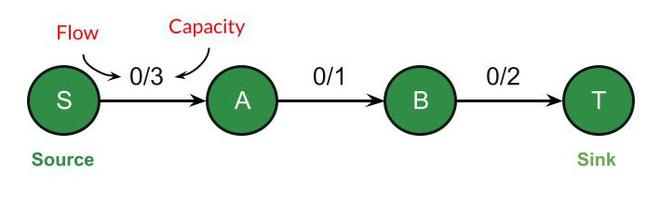We can push flow only downhill, that is, from a vertex at a greater height to one at a lower height. Initially, the source is at a height of V (number of vertices) and the sink is at a height of 0. The height of all the other vertices is 0 and increases as the algorithm progresses. First, we send as much flow as we can from the source to all of its intermediate vertices, where it is stored in their reservoir.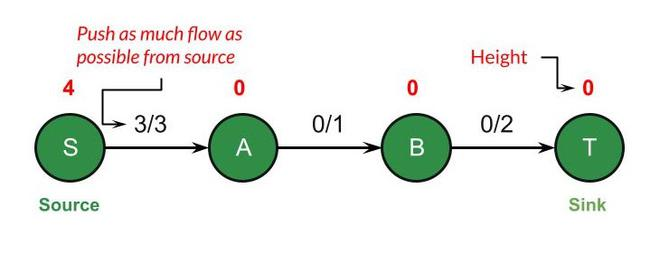Now all the intermediate vertices of the source are overflowing. We can not push flow forward since they will be located at a height 0. In order to send flow forward, we must increase their height by 1.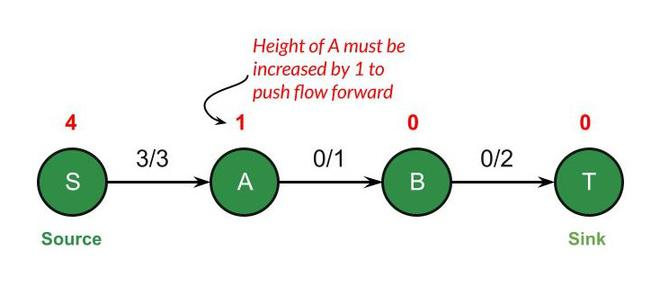The process continues until we reach the sink.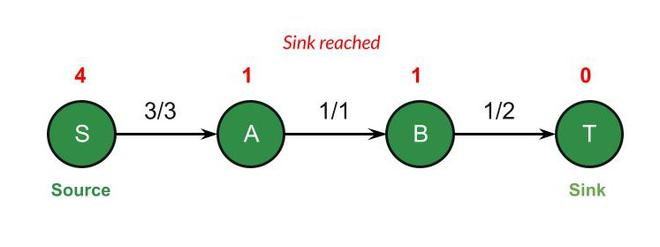In the end, we empty all the excess fluid stored in any of the vertex reservoirs (if any) by sending flow back to the source. The flow obtained then will be maximum flow.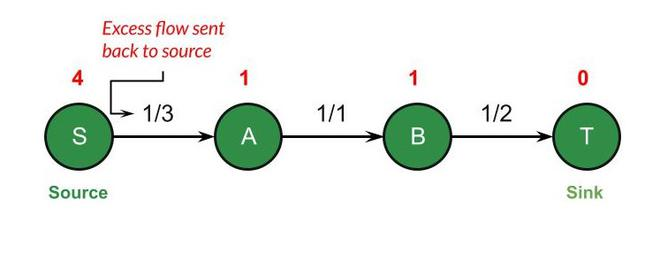Operations

1. Push: Push operation is applied on an overflowing vertex to push the flow forward.  The algorithm finds adjacent vertices of u which are at a lower height than u. For each such adjacent vertex, it sends the maximum possible flow, which is the minimum of excess flow at u and capacity of the edge connecting u with v.
2. Relabel: Relabel operation is applied on an overflowing vertex u to increase its height. The algorithm finds adjacent vertex v of u which has the minimum height. It then updates
```*** QuickLaTeX cannot compile formula:

*** Error message:
Error: Nothing to show, formula is empty
```

Algorithm

The generic push-relabel algorithm uses an initializepre flow function. The function is presented below followed by the algorithm.

Initialize-Preflow

1. initialize height and excess flow of every vertex to 0

2. set height of source to number of vertices

3. initialize flow of each edge to 0

4. for each adjacent vertex of source, set its flow and excess flow to capacity of edge connecting them

Generic-Push-Relabel

1. Initialize-Preflow

2. while there exists an applicable push or relabel operation

3. select an applicable push or relabel operation and perform it

4. return flow

FIFO Push-Relabel vs Push-Relabel

FIFO push relabel is an optimization in the original push relabel. Instead of spending linear time finding the overflowing vertex, we organise all the overflowing vertices in a queue. Doing so allows us to find an overflowing vertex in constant time. This reduces the time complexity to O(V3) from O(V2E).

Implementation of FIFO Push-Relabel Algorithm

Example Problem Statement: In below code, a directed graph has been encapsulated in a class DirectedGraph which has been implemented first. It contains a nested class Vertex which encapsulates the edge of the graph. Consider the graph given below –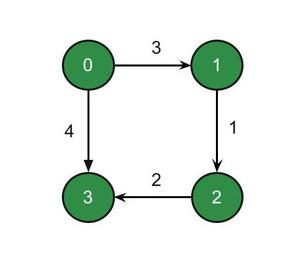The edge from 0 -> 1 will be encapsulated by a vertex object V(1, 3) where 1 is the destination and 3 is the weight. It will be stored at index 0 of adjacency matrix. Here is the adjacency matrix of the above graph.

```0: [[1, 3], [3, 4]]
1: [[2, 1]]
2: [[3, 2]]
3: []```

## Java

 `import` `java.util.ArrayList;` `import` `java.util.LinkedList;`   `// DirectedGraph class explained above` `class` `DirectedGraph {` `    ``public` `static` `class` `Vertex {`   `        ``// number of the end vertex` `        ``// weight or capacity` `        ``// associated with the edge`   `        ``Integer i;` `        ``Integer w;`   `        ``public` `Vertex(Integer i, Integer w)` `        ``{` `            ``this``.i = i;` `            ``this``.w = w;` `        ``}` `    ``}`   `    ``final` `ArrayList > adjacencyList;` `    ``int` `vertices;`   `    ``public` `DirectedGraph(``int` `vertices)` `    ``{` `        ``this``.vertices = vertices;`   `        ``adjacencyList = ``new` `ArrayList<>(vertices);` `        ``for` `(``int` `i = ``0``; i < vertices; i++)` `            ``adjacencyList.add(``new` `ArrayList<>());` `    ``}`   `    ``public` `void` `addEdge(Integer u, Integer v,` `                        ``Integer weight)` `    ``{` `        ``adjacencyList.get(u)` `            ``.add(``new` `Vertex(v, weight));` `    ``}`   `    ``boolean` `hasEdge(``int` `u, ``int` `v)` `    ``{` `        ``if` `(u >= vertices)` `            ``return` `false``;`   `        ``for` `(Vertex vertex : adjacencyList.get(u))` `            ``if` `(vertex.i == v)` `                ``return` `true``;` `        ``return` `false``;` `    ``}`   `    ``// Returns null if no edge` `    ``// is found between u and v` `    ``DirectedGraph.Vertex getEdge(``int` `u, ``int` `v)` `    ``{` `        ``for` `(DirectedGraph.Vertex vertex :` `             ``adjacencyList.get(u))` `            ``if` `(vertex.i == v)` `                ``return` `vertex;`   `        ``return` `null``;` `    ``}` `}`   `public` `class` `MaxFlow {` `    ``private` `final` `int` `source;` `    ``private` `final` `int` `sink;` `    ``private` `final` `DirectedGraph graph;`   `    ``private` `DirectedGraph residualGraph;`   `    ``public` `MaxFlow(DirectedGraph graph,` `                   ``int` `source,` `                   ``int` `sink)` `    ``{` `        ``this``.graph = graph;` `        ``this``.source = source;` `        ``this``.sink = sink;` `    ``}`   `    ``private` `void` `initResidualGraph()` `    ``{` `        ``residualGraph` `            ``= ``new` `DirectedGraph(graph.vertices);`   `        ``// Construct residual graph` `        ``for` `(``int` `u = ``0``; u < graph.vertices; u++) {`   `            ``for` `(DirectedGraph.Vertex v :` `                 ``graph.adjacencyList.get(u)) {`   `                ``// If forward edge already` `                ``// exists, update its weight` `                ``if` `(residualGraph.hasEdge(u, v.i))` `                    ``residualGraph.getEdge(u, v.i).w` `                        ``+= v.w;`   `                ``// In case it does not` `                ``// exist, create one` `                ``else` `                    ``residualGraph.addEdge(u, v.i, v.w);`   `                ``// If backward edge does` `                ``// not already exist, add it` `                ``if` `(!residualGraph.hasEdge(v.i, u))` `                    ``residualGraph.addEdge(v.i, u, ``0``);` `            ``}` `        ``}` `    ``}`   `    ``public` `int` `FIFOPushRelabel()` `    ``{` `        ``initResidualGraph();`   `        ``LinkedList queue` `            ``= ``new` `LinkedList<>();`   `        ``// Step 1: Initialize pre-flow`   `        ``// to store excess flow` `        ``int``[] e = ``new` `int``[graph.vertices];`   `        ``// to store height of vertices` `        ``int``[] h` `            ``= ``new` `int``[graph.vertices];`   `        ``boolean``[] inQueue` `            ``= ``new` `boolean``[graph.vertices];`   `        ``// set the height of source to V` `        ``h = graph.vertices;`   `        ``// send maximum flow possible` `        ``// from source to all its adjacent vertices` `        ``for` `(DirectedGraph.Vertex v :` `             ``graph.adjacencyList.get(source)) {` `            ``residualGraph.getEdge(source, v.i).w = ``0``;` `            ``residualGraph.getEdge(v.i, source).w = v.w;`   `            ``// update excess flow` `            ``e[v.i] = v.w;`   `            ``if` `(v.i != sink) {` `                ``queue.add(v.i);` `                ``inQueue[v.i] = ``true``;` `            ``}` `        ``}`   `        ``// Step 2: Update the pre-flow` `        ``// while there remains an applicable` `        ``// push or relabel operation` `        ``while` `(!queue.isEmpty()) {`   `            ``// vertex removed from` `            ``// queue in constant time` `            ``int` `u = queue.removeFirst();` `            ``inQueue[u] = ``false``;`   `            ``relabel(u, h);` `            ``push(u, e, h, queue, inQueue);` `        ``}`   `        ``return` `e[sink];` `    ``}`   `    ``private` `void` `relabel(``int` `u, ``int``[] h)` `    ``{` `        ``int` `minHeight = Integer.MAX_VALUE;`   `        ``for` `(DirectedGraph.Vertex v :` `             ``residualGraph.adjacencyList.get(u)) {` `            ``if` `(v.w > ``0``)` `                ``minHeight = Math.min(h[v.i],` `                                     ``minHeight);` `        ``}`   `        ``h[u] = minHeight + ``1``;` `    ``}`   `    ``private` `void` `push(``int` `u, ``int``[] e, ``int``[] h,` `                      ``LinkedList queue,` `                      ``boolean``[] inQueue)` `    ``{` `        ``for` `(DirectedGraph.Vertex v :` `             ``residualGraph.adjacencyList.get(u)) {` `            ``// after pushing flow if` `            ``// there is no excess flow,` `            ``// then break` `            ``if` `(e[u] == ``0``)` `                ``break``;`   `            ``// push more flow to` `            ``// the adjacent v if possible` `            ``if` `(v.w > ``0` `&& h[v.i] < h[u]) {` `                ``// flow possible` `                ``int` `f = Math.min(e[u], v.w);`   `                ``v.w -= f;` `                ``residualGraph.getEdge(v.i, u).w += f;`   `                ``e[u] -= f;` `                ``e[v.i] += f;`   `                ``// add the new overflowing` `                ``// immediate vertex to queue` `                ``if` `(!inQueue[v.i] && v.i != source` `                    ``&& v.i != sink) {` `                    ``queue.add(v.i);` `                    ``inQueue[v.i] = ``true``;` `                ``}` `            ``}` `        ``}`   `        ``// if after sending flow to all the` `        ``// intermediate vertices, the` `        ``// vertex is still overflowing.` `        ``// add it to queue again` `        ``if` `(e[u] != ``0``) {` `            ``queue.add(u);` `            ``inQueue[u] = ``true``;` `        ``}` `    ``}`   `    ``public` `static` `void` `main(String[] args)` `    ``{` `        ``final` `int` `vertices = ``6``;` `        ``final` `int` `source = ``0``;` `        ``final` `int` `sink = ``5``;`   `        ``DirectedGraph dg` `            ``= ``new` `DirectedGraph(vertices);`   `        ``dg.addEdge(``0``, ``1``, ``16``);` `        ``dg.addEdge(``0``, ``2``, ``13``);` `        ``dg.addEdge(``1``, ``2``, ``10``);` `        ``dg.addEdge(``2``, ``1``, ``4``);` `        ``dg.addEdge(``1``, ``3``, ``12``);` `        ``dg.addEdge(``3``, ``2``, ``9``);` `        ``dg.addEdge(``2``, ``4``, ``14``);` `        ``dg.addEdge(``4``, ``5``, ``4``);` `        ``dg.addEdge(``4``, ``3``, ``7``);` `        ``dg.addEdge(``3``, ``5``, ``20``);`   `        ``MaxFlow maxFlow` `            ``= ``new` `MaxFlow(` `                ``dg, source, sink);` `        ``System.out.println(` `            ``"Max flow: "` `            ``+ maxFlow.FIFOPushRelabel());` `    ``}` `}`

## C#

 `using` `System;` `using` `System.Collections.Generic;`   `// DirectedGraph class explained above` `class` `DirectedGraph` `{` `  ``public` `class` `Vertex ` `  ``{`   `    ``// number of the end vertex` `    ``// weight or capacity` `    ``// associated with the edge`   `    ``public` `int` `i;` `    ``public` `int` `w;`   `    ``public` `Vertex(``int` `i, ``int` `w)` `    ``{` `      ``this``.i = i;` `      ``this``.w = w;` `    ``}` `  ``}`   `  ``readonly` `public`  `List > adjacencyList;` `  ``public` `int` `vertices;`   `  ``public` `DirectedGraph(``int` `vertices)` `  ``{` `    ``this``.vertices = vertices;`   `    ``adjacencyList = ``new` `List >(vertices);` `    ``for` `(``int` `i = 0; i < vertices; i++)` `      ``adjacencyList.Add(``new` `List());` `  ``}`   `  ``public` `void` `addEdge(``int` `u, ``int` `v,` `                      ``int` `weight)` `  ``{` `    ``adjacencyList[u]` `      ``.Add(``new` `Vertex(v, weight));` `  ``}`   `  ``public` `bool` `hasEdge(``int` `u, ``int` `v)` `  ``{` `    ``if` `(u >= vertices)` `      ``return` `false``;`   `    ``foreach` `(Vertex vertex ``in` `adjacencyList[u])` `      ``if` `(vertex.i == v)` `        ``return` `true``;` `    ``return` `false``;` `  ``}`   `  ``// Returns null if no edge` `  ``// is found between u and v` `  ``public` `DirectedGraph.Vertex getEdge(``int` `u, ``int` `v)` `  ``{` `    ``foreach` `(DirectedGraph.Vertex vertex ``in` `             ``adjacencyList[u])` `      ``if` `(vertex.i == v)` `        ``return` `vertex;`   `    ``return` `null``;` `  ``}` `}`   `public` `class` `MaxFlow {` `  ``private` `readonly` `int` `source;` `  ``private` `readonly` `int` `sink;` `  ``private` `readonly` `DirectedGraph graph;`   `  ``private` `DirectedGraph residualGraph;`   `  ``MaxFlow(DirectedGraph graph,` `          ``int` `source,` `          ``int` `sink)` `  ``{` `    ``this``.graph = graph;` `    ``this``.source = source;` `    ``this``.sink = sink;` `  ``}`   `  ``private` `void` `initResidualGraph()` `  ``{` `    ``residualGraph` `      ``= ``new` `DirectedGraph(graph.vertices);`   `    ``// Construct residual graph` `    ``for` `(``int` `u = 0; u < graph.vertices; u++) {`   `      ``foreach` `(DirectedGraph.Vertex v ``in` `               ``graph.adjacencyList[u]) {`   `        ``// If forward edge already` `        ``// exists, update its weight` `        ``if` `(residualGraph.hasEdge(u, v.i))` `          ``residualGraph.getEdge(u, v.i).w` `          ``+= v.w;`   `        ``// In case it does not` `        ``// exist, create one` `        ``else` `          ``residualGraph.addEdge(u, v.i, v.w);`   `        ``// If backward edge does` `        ``// not already exist, add it` `        ``if` `(!residualGraph.hasEdge(v.i, u))` `          ``residualGraph.addEdge(v.i, u, 0);` `      ``}` `    ``}` `  ``}`   `  ``public` `int` `FIFOPushRelabel()` `  ``{` `    ``initResidualGraph();`   `    ``List<``int``> queue` `      ``= ``new` `List<``int``>();`   `    ``// Step 1: Initialize pre-flow`   `    ``// to store excess flow` `    ``int``[] e = ``new` `int``[graph.vertices];`   `    ``// to store height of vertices` `    ``int``[] h` `      ``= ``new` `int``[graph.vertices];`   `    ``bool``[] inQueue` `      ``= ``new` `bool``[graph.vertices];`   `    ``// set the height of source to V` `    ``h = graph.vertices;`   `    ``// send maximum flow possible` `    ``// from source to all its adjacent vertices` `    ``foreach` `(DirectedGraph.Vertex v ``in` `             ``graph.adjacencyList) {` `      ``residualGraph.getEdge(source, v.i).w = 0;` `      ``residualGraph.getEdge(v.i, source).w = v.w;`   `      ``// update excess flow` `      ``e[v.i] = v.w;`   `      ``if` `(v.i != sink) {` `        ``queue.Add(v.i);` `        ``inQueue[v.i] = ``true``;` `      ``}` `    ``}`   `    ``// Step 2: Update the pre-flow` `    ``// while there remains an applicable` `    ``// push or relabel operation` `    ``while` `(queue.Count!=0) {`   `      ``// vertex removed from` `      ``// queue in constant time` `      ``int` `u = queue;` `      ``queue.RemoveAt(0);` `      ``inQueue[u] = ``false``;`   `      ``relabel(u, h);` `      ``push(u, e, h, queue, inQueue);` `    ``}`   `    ``return` `e[sink];` `  ``}`   `  ``private` `void` `relabel(``int` `u, ``int``[] h)` `  ``{` `    ``int` `minHeight = ``int``.MaxValue;`   `    ``foreach` `(DirectedGraph.Vertex v ``in` `             ``residualGraph.adjacencyList[u]) {` `      ``if` `(v.w > 0)` `        ``minHeight = Math.Min(h[v.i],` `                             ``minHeight);` `    ``}`   `    ``h[u] = minHeight + 1;` `  ``}`   `  ``private` `void` `push(``int` `u, ``int``[] e, ``int``[] h,` `                    ``List<``int``> queue,` `                    ``bool``[] inQueue)` `  ``{` `    ``foreach` `(DirectedGraph.Vertex v ``in` `             ``residualGraph.adjacencyList[u])` `    ``{`   `      ``// after pushing flow if` `      ``// there is no excess flow,` `      ``// then break` `      ``if` `(e[u] == 0)` `        ``break``;`   `      ``// push more flow to` `      ``// the adjacent v if possible` `      ``if` `(v.w > 0 && h[v.i] < h[u]) {` `        ``// flow possible` `        ``int` `f = Math.Min(e[u], v.w);`   `        ``v.w -= f;` `        ``residualGraph.getEdge(v.i, u).w += f;`   `        ``e[u] -= f;` `        ``e[v.i] += f;`   `        ``// add the new overflowing` `        ``// immediate vertex to queue` `        ``if` `(!inQueue[v.i] && v.i != source` `            ``&& v.i != sink) {` `          ``queue.Add(v.i);` `          ``inQueue[v.i] = ``true``;` `        ``}` `      ``}` `    ``}`   `    ``// if after sending flow to all the` `    ``// intermediate vertices, the` `    ``// vertex is still overflowing.` `    ``// add it to queue again` `    ``if` `(e[u] != 0) {` `      ``queue.Add(u);` `      ``inQueue[u] = ``true``;` `    ``}` `  ``}`   `  ``public` `static` `void` `Main(String[] args)` `  ``{` `    ``int` `vertices = 6;` `    ``int` `source = 0;` `    ``int` `sink = 5;`   `    ``DirectedGraph dg` `      ``= ``new` `DirectedGraph(vertices);`   `    ``dg.addEdge(0, 1, 16);` `    ``dg.addEdge(0, 2, 13);` `    ``dg.addEdge(1, 2, 10);` `    ``dg.addEdge(2, 1, 4);` `    ``dg.addEdge(1, 3, 12);` `    ``dg.addEdge(3, 2, 9);` `    ``dg.addEdge(2, 4, 14);` `    ``dg.addEdge(4, 5, 4);` `    ``dg.addEdge(4, 3, 7);` `    ``dg.addEdge(3, 5, 20);`   `    ``MaxFlow maxFlow` `      ``= ``new` `MaxFlow(` `      ``dg, source, sink);` `    ``Console.WriteLine(` `      ``"Max flow: "` `      ``+ maxFlow.FIFOPushRelabel());` `  ``}` `}`   `// This code is contributed by 29AjayKumar`

Output

`Max flow: 23`

Time Complexity: O(V3)

My Personal Notes arrow_drop_up
Recommended Articles
Page :